Next: 4.1.2 Phase Transformation Properties Up: 4.1 Principles of Fourier Previous: 4.1 Principles of Fourier

## 4.1.1 Scalar Diffraction Theory

Light is an electromagnetic wave with coupled electric and magnetic fields traveling through space. In a homogeneous isotropic medium, like free space or a lens with constant refractive index, the electric and magnetic field vectors form a right-handed orthogonal triad with the direction of propagation. Disregarding polarization, the field can be described by a scalar function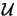(x;t) representing either the electric or the magnetic field amplitude. As the light used in microlithography exhibits a strong monochromacity (cf. Section 2.3.1) the time dependence of the field is a harmonic one and can thus be explicitly written as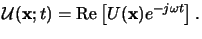(4.1)

Here,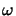denotes the angular frequency of the light, and the complex-valued amplitude or phasor  U(x) depends on the spatial coordinates x = (x  y  z)T only. If both quantities(x;t) and U(x) represent an optical wave, they must satisfy the Helmholtz equationa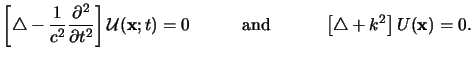(4.2)

The Helmholtz equation directly follows from the Maxwell equations under the condition of a homogeneous medium and in absence of sources. The quantity k is termed the wavenumber or propagation constant of the medium and is related to the light velocity c, the angular frequency, and the vacuum wavelength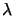byb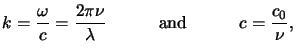(4.3)

whereby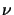is the refractive index of the medium, e.g., in vacuum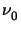= 1.

The complex disturbance  U(x) at any observation point xo in space can be computed from the Helmholtz equation (4.2) with the help of Green's theorem.c If a unit-amplitude spherical wave expanding about the observation point x0 is chosen as Green's function, i.e.,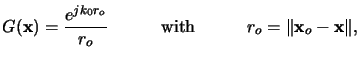(4.4)

the so-called integral theorem of Helmholtz and Kirchhoff is obtained [11, p. 377]: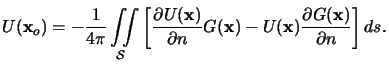(4.5)

This relation plays a central role in the derivation of the scalar theory of diffraction, for it allows the field U(x) at an observation point xo to be expressed in terms of the boundary values'' on any closed surface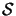surrounding xo.

The integral theorem of (4.5) can readily be used to study diffraction effects occurring at a plane object. Therefore the integration surfaceis segmented into three disjoint parts, i.e.,=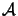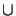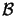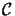. The boundaryis chosen across the object location in the screen plane,is the opaque part of the screen, andis a half-sphere containing the observation point xo. A graphical illustration of this specific choice is given in Figure 4.1. The solution of the diffraction problem is found by specifying Kirchhoff boundary conditions onand, and the Sommerfeld radiation condition on: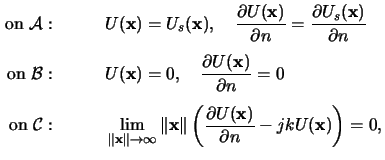whereby Us(x) is the field incident on the screen. The Kirchhoff boundary conditions are an idealization to the real field distribution on the screen by assuming that the field and its derivative acrossis exactly the same as they would be in the absence of the screen. In the geometrical shadow acrossthe field is simply set to zero. Although these assumptions are reasonable, they fail in the immediate neighborhood of the rim of the opening. Thus, the range of validity is related to the wavelengthand the geometrical dimension w of the opening (cf. Figure 4.1). The Sommerfeld radiation condition onis satisfied, if the disturbance U(x) vanishes at least as fast as a spherical wave. Since the illuminating light Us(x) invariably consists of a linear combination of spherical waves, this requirement is always fulfilled.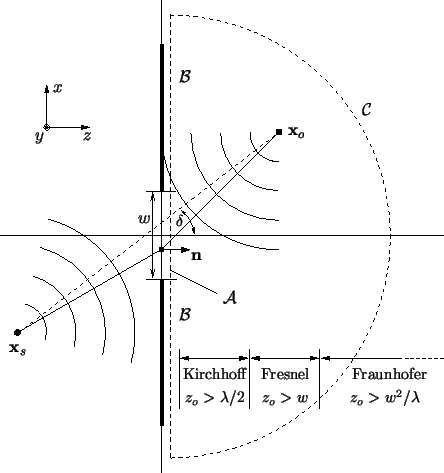Inserting the boundary conditions of (4.6) into the integral theorem (4.5) shows that only the illuminating light Us(x) across the openingcontributes to the integral, i.e.,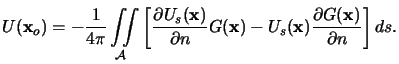(4.6)

We further assume that the aperture is illuminated with a single expanding spherical wave arising from a point source located in xs, a distance rs away from the screen (cf. Figure 4.1),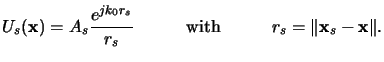(4.7)

Hence, both Green's function in (4.4) and the incident disturbance in (4.8) have the shape of spherical waves. A further simplification is obtained by noting that the distances from the screen to the observation point and the location of the point source, ro and rs, respectively, are many optical wavelengths. Therefore the following approximations hold for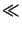ro, rs:d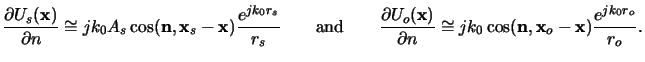(4.8)

Insertion of these approximations into (4.7) yields together with (4.4) and (4.8) the Fresnel-Kirchhoff diffraction formula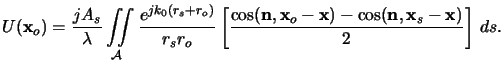(4.9)

The term in square brackets is called obliquity or inclination factor and was first introduced by Fresnel based on heuristic arguments, when he combined Huygens' envelope construction with Young's principle of interference. The mathematical sound derivation described above was later developed by Kirchhoff. An interesting property of the diffraction formula (4.10) is its symmetry with respect to the source and observation point, a result that is sometimes referred to as the reciprocity theorem of Helmholtz.

For our purposes we rewrite (4.10) by introducing a general linear relationship between the exciting field Us(x, y) at the screen and the resulting diffraction pattern U(xo, yo)e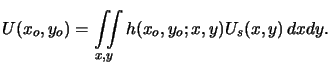(4.10)

Note that we are always interested in field values lying in certain planes parallel to the screen. Therefore, only the dependence on the lateral x- and y-coordinate is explicitly written, whereas the vertical position is indicated by subscripts. The integration kernel h(x, y;xo, yo) of the superposition integral in (4.11) is given by (cf. (4.8) and (4.10))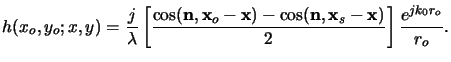(4.11)

Depending on the distance zo between the observation point xo and the screen, the kernel h(xo, yo;x, y) can be simplified. The denominator ro is approximated by zo provided that zo is much larger than the lateral extent w of the object. For the same reason and by assuming that the source as well as the observation point are located near the optical axis, the inclination factor is approximately given by cos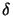(cf. Appendix A). As shown in Figure 4.1 the anglelies between the line from source to observation point and the normal vector n. The approximation of the exponential factor is more involved, since ro is multiplied by the very large wavenumber k0. Hence, a truncation of Taylor series expansions is employed,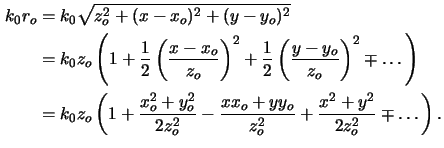• Fresnel approximations are obtained by truncating the Taylor series after the first term, i.e.,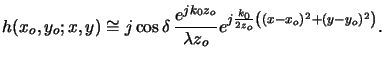(4.12)

The resulting integral relation (4.11) involves Fresnel's integrals.f Due to the square dependence on the integration variables (x, y), no analytical solution exists. Tabulated values can be found in, e.g., [115, ch. 7]. The approximation (4.14) holds for (2zo)3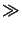k0[(x - xo)2 + (y - yo)2]2max or simply zow.
• Fraunhofer approximations additionally neglect the square dependence on the integration variables (x, y) resulting in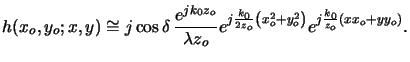(4.13)

The integral relation (4.11) corresponds now to a Fourier transform, i.e., the dependence on the integration variables is a linear one. Efficient numerical techniques like FFT can be used for evaluation. The range of validity of (4.15) is given by 2zok(x2 + y2)max or zw2/.

#### Footnotes

... a
The symbol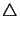denotes the Laplace operator, i.e.,=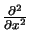+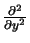+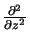.
... byb
The quantity c0 is the vacuum light velocity and has a value of c02.998  108 m/s.
... theorem.c
Green's theorem states that if a complex-valued function U(x) is continuous and has continuous single-valued first and second partial derivatives inside a volume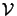surrounded by a closed surface, the following relation holds: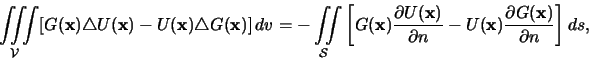whereby the so-called Green's function  G(x) is any other function with the same continuity requirements as U(x) and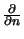= n . grad signifies a partial derivative in the inward normal direction n at each point on.
...:d
Forr and thus 1/rk0 we get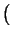ejk0r/r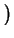= cos(n, x)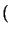jk0 - 1/r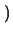ejk0r/r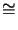jk0cos(n, x)ejk0r/r.
...)e
All integrations and sums go from -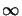tounless specified otherwise.
... integrals.f
Integrals of the type C(w) =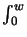cos(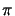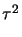/2) d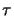and S(w) =sin(/2) dare referred to as Fresnel's integrals [115, ch. 7].Next: 4.1.2 Phase Transformation Properties Up: 4.1 Principles of Fourier Previous: 4.1 Principles of Fourier
Heinrich Kirchauer, Institute for Microelectronics, TU Vienna
1998-04-17